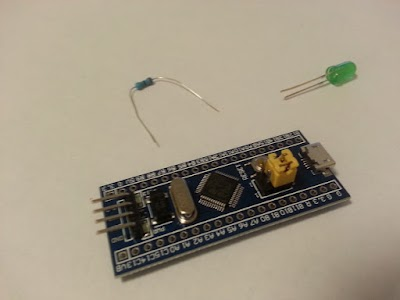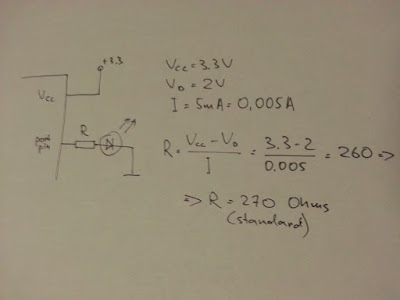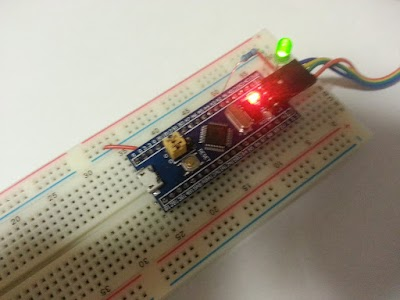# Current limiting resistor for an LED

I am a beginner in electronics, and just learned that LEDs and microcontroller’s I/O ports are so sensitive … Thanks Mr. Ohm whose law saves us!A current limiting resistor should be used for driving an LED from a microcontroller’s I/O pin to limit the current through the LED. The max current value for an LED can be found in its datasheet. It is usually around 20mA. Another reason for using a current limiting resistor is that microcontroller’s I/O ports have a maximum I/O current rating. It can be found in a datasheet for microcontroller you use. For example, it is 25mA for STM32F103 (see “Table 7. Current characteristics” in datasheet).

To calculate the value for a current limiting resistor, you just need to use your favorite Ohm’s law. Here is a circuit and calculations.“Vcc” is a power supply voltage (it is 3.3V in case of STM32F103). We assume that high level input voltage of I/O pin is equal to Vcc (which is not actually true, see section “5.3.13 I/O port characteristics” in datasheet).
The desired current through the LED should be less than its max current value (usually around 20mA). I used 5mA because I wanted just an indication, not lighting.
The formula told me that I should be happy with 260 Ohms. The closest standard resistor is 270 Ohms.By the way, there are lots of online calculators which you can use if you don’t want to waste paper. For example, see http://www.ohmslawcalculator.com/led-resistor-calculator
Have a next day!

If you have found a spelling error, please, notify us by selecting that text and pressing Ctrl+Enter.